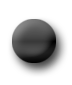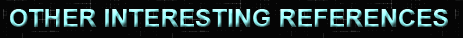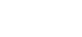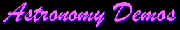• <== animations of concepts in astronomy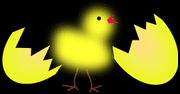• <== experience the connection between math and physics for yourself. which came first? "The Dance of Math and Physics"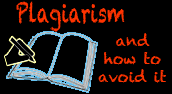• <== learn how to avoid the most common forms of plagiarism• <== if you have it, click on it and get rid of it!• <== What science is and is not, written by a research scientist (me!)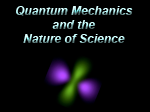• <== What is quantum mechanics and how does it fit into our paradigm of science?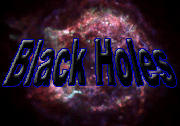• <== find out what they are, how they work, and why there's a supermassive black hole at the center of the Milky Way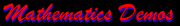• <== visualize the abstract!• <== is that really the color of space clouds, stars, and galaxies? find out.• <== VSU's Fossil Museum— see animals that lived millions of years ago. • See a list of upcoming Public Planetarium Shows • Visit the VSU ASTRONOMY page • Visit the VSU PHYSICS page http://www.spaceweather.com • <== Keep track of the sun http://apod.nasa.gov • <== Astronomy Picture of the Day http://www.nasa.gov • <== NASA mission site http://www.stsci.org • <== Hubble Space Telescope Site https://www.youtube.com • <== Why Bother Learning Math